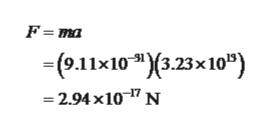An electron is a subatomic particle (m = 9.11 x 10-31 kg) that is subject to electric forces. An electron moving in the +x direction accelerates from an initial velocity of +7.11 x 105 m/s to a final velocity of 2.21 x 106 m/s while traveling a distance of 0.0678 m. The electron's acceleration is due to two electric forces parallel to the x axis:  = 7.82 x 10-17 N, and , which points in the -x direction. Find the magnitudes of (a) the net force acting on the electron and (b) the electric force

Question

An electron is a subatomic particle (m = 9.11 x 10-31 kg) that is subject to electric forces. An electron moving in the +x direction accelerates from an initial velocity of +7.11 x 105 m/s to a final velocity of 2.21 x 106 m/s while traveling a distance of 0.0678 m. The electron's acceleration is due to two electric forces parallel to the x axis:  = 7.82 x 10-17 N, and , which points in the -x direction. Find the magnitudes of (a) the net force acting on the electron and (b) the electric force

Step 1

The acceleration of the of the electron is,

Step 2

a)

The required net ...help_outlineImage TranscriptioncloseF ma - (о1х10 "(3.23х10") -2.94 x10-7 N fullscreen

Want to see the full answer?

See Solution

Want to see this answer and more?

Our solutions are written by experts, many with advanced degrees, and available 24/7

See Solution
Tagged in

Electric Charges and Fields